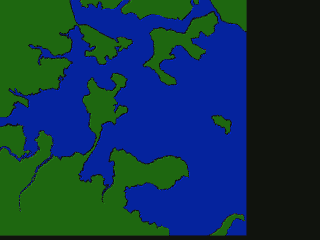# Boston Landfill# Boston Landfill

This is a project I became interested in a long time ago. I have been in Boston for over 12 years, and knew that Boston was built over time from a series of landfill projects. I was interested in creating an app that allowed a user to scrub through time and see what parts of Boston were changed and when.

After a little bit of digging, I found this dataset here, an extremely poor quality GIF. I probably could have emailed the professor for something of higher quality, but since I am always up for a challenge, I wanted to use this to practice a variety of skills:

• Understand GIFs in Python
• Some basic image processing: dilation/erosion, thresholding, smoothing, etc.
• Extract contours/shapes from an image
• Shape manipulations: merging, intersection
• SVG creation
• GeoJSON creation
• Interactive React App with GeoJSON objects
• React App for timelines, and other Interactive elements

## Data

Original data was sourced here, a GIF image: http://www.bc.edu/bc_org/avp/cas/fnart/fa267/sequence.html## Key Lessons Learned

A colored GIF is just a F x M x N x C tensor, where F is the number of frames, M and N are the height and width, and C=3 are the 3 RGB channels.

Easy to read image (and make Greyscale):

from skimage.io import imread
from skimage.color import rgb2gray

image = rgb2gray(imread('img.gif'))

### Basic Image Processing

#### Resizing

from skimage.transform import resize as resize_image

image = resize_image(image, (1000, 1000))

#### Slight blur

from skimage.filters import gaussian

image = gaussian(image, 2)

#### Thresholding

I used threshold_minimum which is described as: The histogram of the input image is computed and smoothed until there are only two maxima. Then the minimum in between is the threshold value.

It is described in more detail here

threshold = threshold_minimum(rimage)

binary = (image > threshold).astype(int)
# binary = binary_dilation(binary)

return binary


#### Erosion/Dilation

from skimage.morphology import binary_dilation, binary_erosion

image = binary_dilation(image)

### Get contours from image

This creates contours where image = 0. Since we created a binary image with thresholding, this is easy.

from skimage import measure

contours = measure.find_contours(image, 0)

### Contour to Shapes

from shapely.geometry import Polygon

polygon = Polygon(c)

### Simplify shapes

p = polygon.simplify(0.5, preserve_topology=True)

### Convert contours to SVG

In order to convert Shapely shapes to SVG, I manually built my own SVG path elements, similar to this:

path = svgwrite.path.Path()
ext_points = list(shape.exterior.coords)

P = [['M', int(ext_points), int(ext_points)]] + [['L', int(x), int(x)] for x in ext_points[1:]] + [['Z']]
[path.push(*x) for x in P]

for interior in shape.interiors:
int_points = list(interior.coords)

P = [['M', int(int_points), int(int_points)]] + [['L', int(x), int(x)] for x in int_points[1:]] + [['Z']]
[path.push(*x) for x in P]

### Convert SVG to Polygons

In order to convert Shapely shapes to SVG, I manually built my own SVG path elements, similar to this:

from shapely.geometry import Polygon, MultiPolygon
from xml.dom import minidom, Node
from svg.path import parse_path as parse_svg_path

svg_doc = minidom.parse("data/svg_out.svg")

def parse_path(path):
points = map(lambda x: (x.start.real, x.start.imag), parse_svg_path(path.getAttribute('d')))
return points

points = parse_path(svg_doc.getElementsByTagName("path"))
Polygon(points)

### Convert Polygons to GeoJSON

from shapely.geometry import mapping
import json

out = {
"type": "FeatureCollection",
"features": [
{
"type": "Feature",
"properties": { "layer": 5, "region": "logan_airport" },
"geometry": mapping(shape)
}
]
}

json.dump(out, open('test.geojson', 'w'))